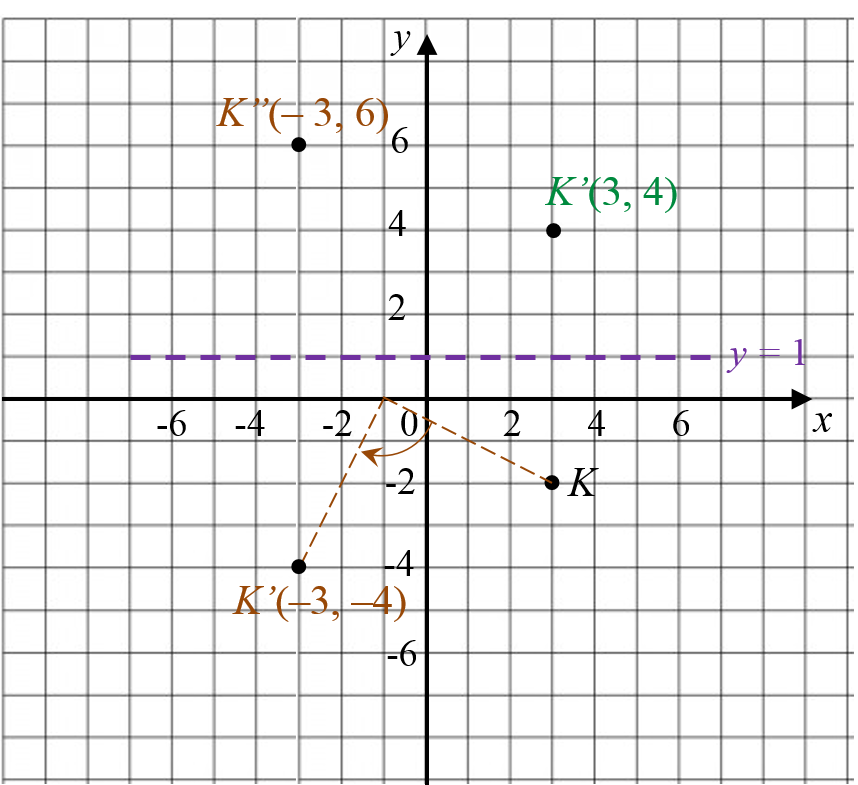# SPM Mathematics 2019, Paper 2 (Question 13)

Question 13:
(a) Diagram 7.1 shows point K on a Cartesian plane.Diagram 7.1

Diagram 7.1 shows point K on a Cartesian plane.
Transformation P is a reflection in the line y = 1.
Transformation R is a clockwise rotation of 90° about the centre (-1,0)
State the coordinates of the image of point K under each of the following transformations:
(i) P,
(ii) PR.
[3 marks]

(b) Diagram 7.2 shows three pentagons EFGHJ, KLMNP, and KQRST, drawn on a Cartesian plane.Diagram 7.2

(i) Pentagon KQRST is the image of pentagon EFGHJ under the combined transformation VU.
Describe, in full, the transformation:
(a) U(b) V.

(ii) It is given that the pentagon KQRST represents a region of area 288 m2.
Calculate the area, in m2, of the shaded region.
[9 marks].

Solution:
(a)(a)(i)
Point K (3, –2) is reflected in the line y = 1.
K
(3, –2) → PK’ (3, 4).
Coordinates of image are (3, 4).

(a)(ii)
K (3, –2) → RK’ (–3, –4) → PK’’ (–3, 6).
Coordinates of image are (–3, 6).

(b)(b)(i)(a)
U: 90o rotation in clockwise direction at centre (–3, 3).

(b)(i)(a)
V: enlargement at centre K(–1, 8) with scale factor 2.

(b)(ii)
Area of KQRST = (scale factor)2 × Area of KLMNP
288 = 22 × Area of KLMNP
Area of KLMNP = 72 m2